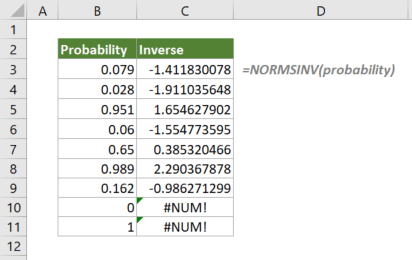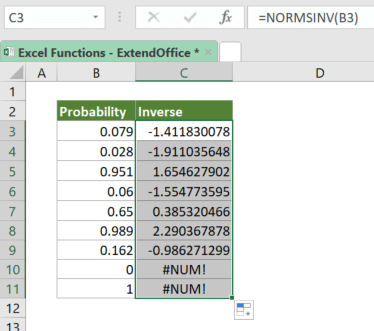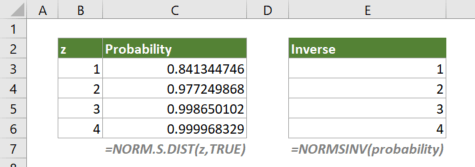## Excel NORMSINV Function

The NORMSINV calculates and returns the inverse of the standard normal cumulative distribution that has an arithmetic mean of 0 and standard deviation of 1 with a given probability.

Note: NORMSINV has been replaced with NORM.S.INV. Although NORMSINV is still available in current versions of Excel, you should consider using the new function from now on, because NORMSINV may not be available in Excel’s future versions.#### Syntax

=NORMSINV(probability)

#### Arguments

• probability (required): The probability corresponding to the normal distribution. It is the value at which you want to evaluate the inverse function.

#### Return Value

The NORMSINV function returns a numeric value.

#### Function Notes

• The #VALUE! error occurs if probability is nonnumeric.
• The #NUM! error occurs if probability ≤ 0 or probability ≥ 1.

#### Example

To get the inverse of the standard normal cumulative distribution with the probabilities as shown in the screenshot below, please copy or enter the formula below in the top cell C3, and press Enter to get the first result:

=NORMSINV(B3)

√ Note: After entering the formula, drag the fill handle down to apply the formula to the below cells.Note that for the normal cumulative distribution (probability) that is calculated with NORM.S.DIST(z, TRUE), the NORMSINV function will return the z value based on the probability result. See screenshot:#### Related functions

Excel NORM.S.INV Function

The NORM.S.INV calculates and returns the inverse of the standard normal cumulative distribution that has an arithmetic mean of 0 and standard deviation of 1 with a given probability.

Excel NORM.INV Function

The NORM.INV calculates and returns the inverse of the normal cumulative distribution for the given arithmetic mean and standard deviation.

Excel NORM.DIST Function

The NORM.DIST calculates and returns the cumulative distribution function or probability density function of a value for the given arithmetic mean and standard deviation.

Excel NORM.S.DIST Function

The NORM.S.DIST calculates and returns the standard normal cumulative distribution function or probability density function of a value for an arithmetic mean of 0 and standard deviation of 1.

### The Best Office Productivity Tools

#### Kutools for Excel - Helps You To Stand Out From Crowd

 Popular Features: Find, Highlight or Identify Duplicates  |  Delete Blank Rows  |  Combine Columns or Cells without Losing Data  |  Round without Formula ... Super VLookup: Multiple Criteria  |  Multiple Value  |  Across Multi-Sheets  |  Fuzzy Lookup... Adv. Drop-down List: Easy Drop Down List  |  Dependent Drop Down List  |  Multi-select Drop Down List... Column Manager: Add a Specific Number of Columns  |  Move Columns  |  Toggle Visibility Status of Hidden Columns  |  Compare Columns to Select Same & Different Cells ... Featured Features: Grid Focus  |  Design View  |  Big Formula Bar  |  Workbook & Sheet Manager | Resource Library (Auto Text)  |  Date Picker  |  Combine Worksheets  |  Encrypt/Decrypt Cells  |  Send Emails by List  |  Super Filter  |  Special Filter (filter bold/italic/strikethrough...) ... Top 15 Toolsets:  12 Text Tools (Add Text, Remove Characters ...)  |  50+ Chart Types (Gantt Chart ...)  |  40+ Practical Formulas (Calculate age based on birthday ...)  |  19 Insertion Tools (Insert QR Code, Insert Picture from Path ...)  |  12 Conversion Tools (Numbers to Words, Currency Conversion ...)  |  7 Merge & Split Tools (Advanced Combine Rows, Split Excel Cells ...)  |  ... and more

Kutools for Excel Boasts Over 300 Features, Ensuring That What You Need is Just A Click Away...#### Office Tab - Enable Tabbed Reading and Editing in Microsoft Office (include Excel)

• One second to switch between dozens of open documents!
• Reduce hundreds of mouse clicks for you every day, say goodbye to mouse hand.
• Increases your productivity by 50% when viewing and editing multiple documents.
• Brings Efficient Tabs to Office (include Excel), Just Like Chrome, Edge and Firefox.